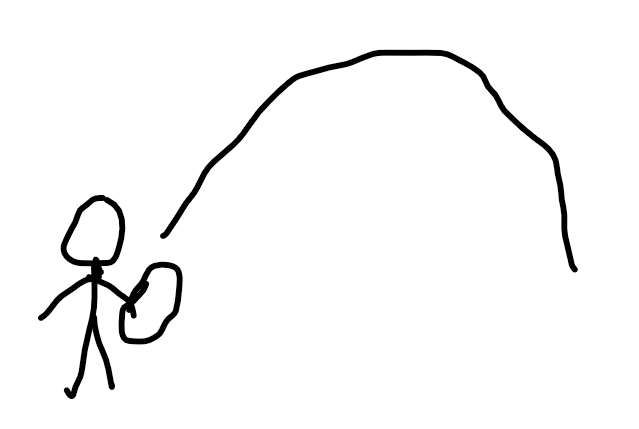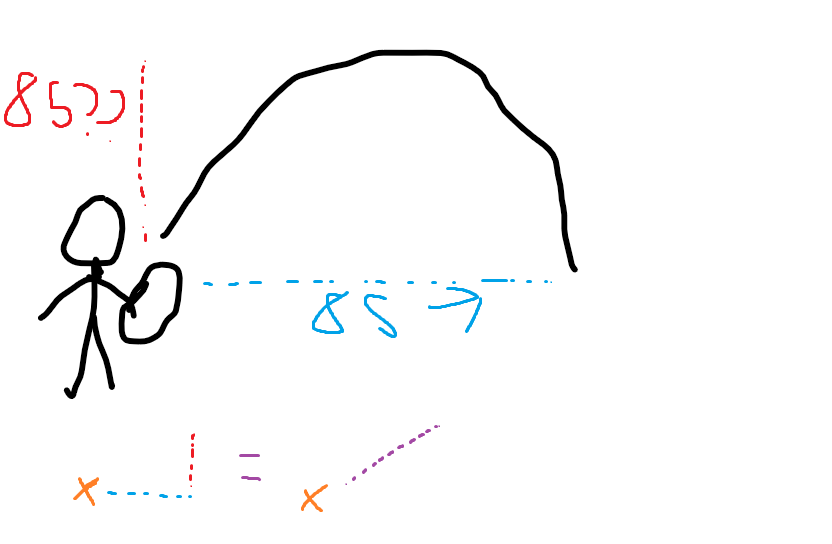# Sword dash ability help

Hey, I am working on a sword and to have a dash ability to it!

My script:

``````local Sword = script.Parent
local db = false

Sword.Activated:Connect(function()
local Humanoid = Sword.Parent:FindFirstChild("Humanoid")
local Root = Sword.Parent:FindFirstChild("HumanoidRootPart")

if db == true or Root:FindFirstChild("BodyVelocity") then return end
db = true

script.Damage.Disabled = false

local BodyVelocity = Instance.new("BodyVelocity",Root)
BodyVelocity:SetAttribute("BV","BV")
BodyVelocity.P = 1500
BodyVelocity.MaxForce = Vector3.new(math.huge,math.huge,math.huge)
BodyVelocity.Velocity = Root.CFrame.lookVector * 85

wait(2.5)

script.Damage.Disabled = true
db = false
end)
``````

The problem is that when the player is not jumping the dash isn’t the same as if the player was jumping the player would dash way more further.

I was wanting a dash like this:Why don’t you apply a force upwards as well?
First make the player jump; Humanoid.Jump

Then you make your body velocity, but with the upwards aswell

``````BodyVelocity.Velocity = (Root.CFrame.lookVector + Root.CFrame.upVector) * 85
``````

I had a typo but I fixed it btw, the code works